CS 351 -Data Organization and Management ( Section: 02)

LectureNotes, Week 4

Prepared by: Seher BAS /Aysan BAYSAL /Esra DALGIC

REPLACEMENT SELECTION SORT

(DUE TO Knuth com of ACM,1965)

Let m be the number of records to be sorted which can be kept in the main memory. Imagine that these memory locations are registers and assume we can mark them as “on” or “off”. Replacement selection can overlap reading, sorting and writing.

The Algorithm:

Step1: The m registers are filled with records from the input to be sorted.

Step2: All registers are put into the “on” state.

Step3: Select the register which has the smallest of all “on” registers.

Step4: Transfer the contents of the selected register to the output (call it as key Y).

Step5: Replace the contents of the selected register by the next input record.

If new record key > Y

Go to step 3

If new record key = =Y

Go to step 4

If new record key < Y

Go to step 6

Step6: Turn the selected key register  “off”.

If all registers are now  “off”

We have completed a sorted substring(run).

We start a new substring group and go to step 2.

Else

Go to step 3

Example: Suppose number of registers is 3 and input keys are 5, 2, 9, 7, 0, 8, 1, 6, 3, 4 ...

Registers                               Output

5 2 9                                       2

5 7 9                                       5

*0 7 9                                     7

*0 8 9                                     8

*0*1 9                                    9

*0*1*6

0 1 6                                       0

3 1 6                                       1

3 4 6                                       3

- 4 6                                        4

- - 6                                        6

- - -

Using Two Disk Drives For Heap Sort: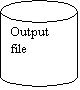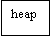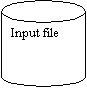Sort time = b* ebt (to create sorted segments)

1.          Create HeapA

2.          Write a block of records of HeapA to disk  read a block of records from input file

3.          Consider the first record of the block read from input file(new key)

If new key < keys written of the records written out

Insert new key to HeapB

Else

Insert new key to HeapA

ONE DISK DRIVE LARGE-MEMORY MERGING

2- way merge, 4-way merge, p-way merge

Assumptions: 2400 MB file 10 MB memory for heap construction.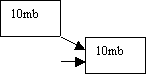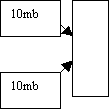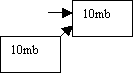Reads 5MB partition of each segment each segment contains seg number of blocks

(4+3)*(r+s)+2*2*seg*ebt

10MB/2400bytes=4167 segments

7*(24,3)+4*4167*0,84=14,2 sec (just to merge two sorted segments four halves of two segments)

Since we have 240 sorted segments

120*14,2=1704 sec = 28,4 min (for the first pass)

 Passes 1 2 3 4 5 6 7 8 Segment size 10 20 40 80 160 Seven 320 one 160 Three 640 one 480 One 1280 one 1120 Number of segment 240 120 60 50 15 8 4 2

Number of passes =[lg240] (for tw0 way merge)

Consider 80 Mb, if we have “nsg ”segment of memory size, we read half of each segment at a time we have 2*nsg pieces.

Cost of one pass = 2*2*(nsg)*(r+s)+2*b*ebt

= 4*240*(24,3)+2*1000000*0,84

=1703 sec=28,4 min/pass

we have 8 passes

Total cost=28(heap sort creates initial run)+8*28,4=28+227=255min=4,25 hours.

# The Four-Way Merge

## Cost of one pass= 2*4*(nsg)*(r+s)+2*b*ebt

=8*240*24,3+2000000*0,84

=28,8 min

[log4240]=4

Total cost= 4*28,8+28=143 min

# The P-Way Merge

## A=Cost of one pass=2*p*(nsg)*(r+s)+2*b*ebt

B=Number of passes=logp (nsg)

Total cost = A*B+ initial sorting time(execution time)

Maximum number of initial runs => p=nsg

A=2*nsg*nsg*(r+s)+2*b*ebt

=2*2402 *24,3+1680000

=74 min.